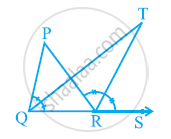Share

In the given figure, the side QR of ΔPQR is produced to a point S. If the bisectors of ∠PQR and ∠PRS meet at point T, then prove that ∠QTR = 1/2∠QPR. - CBSE Class 9 - Mathematics

ConceptAngle Sum Property of a Triangle

Question

In the given figure, the side QR of ΔPQR is produced to a point S. If the bisectors of ∠PQR and ∠PRS meet at point T, then prove that ∠QTR = 1/2∠QPR.Solution

In ΔQTR, ∠TRS is an exterior angle.

∴ ∠QTR + ∠TQR = ∠TRS

∠QTR = ∠TRS − ∠TQR ...........(1)

For ΔPQR, ∠PRS is an external angle.

∴ ∠QPR + ∠PQR = ∠PRS

∠QPR + 2∠TQR = 2∠TRS (As QT and RT are angle bisectors)

∠QPR = 2(∠TRS − ∠TQR)

∠QPR = 2∠QTR [By using equation (1)]

∠QTR = 1/2∠QPR

Is there an error in this question or solution?

APPEARS IN

NCERT Solution for Mathematics Class 9 (2018 to Current)
Chapter 6: Lines and Angles
Ex.6.30 | Q: 6 | Page no. 108

Video TutorialsVIEW ALL 

Solution In the given figure, the side QR of ΔPQR is produced to a point S. If the bisectors of ∠PQR and ∠PRS meet at point T, then prove that ∠QTR = 1/2∠QPR. Concept: Angle Sum Property of a Triangle.
S